Browse by Topic
Related Topics

# New Wolfram Books: Releases from Wolfram Media and Others Featuring the Wolfram Language

The first half of 2020 has brought with it another exciting batch of publications. Wolfram Media has released Conrad Wolfram’s The Math(s) Fix. Keep an eye out for the upcoming third edition of Hands-on Start to Wolfram Mathematica later in 2020.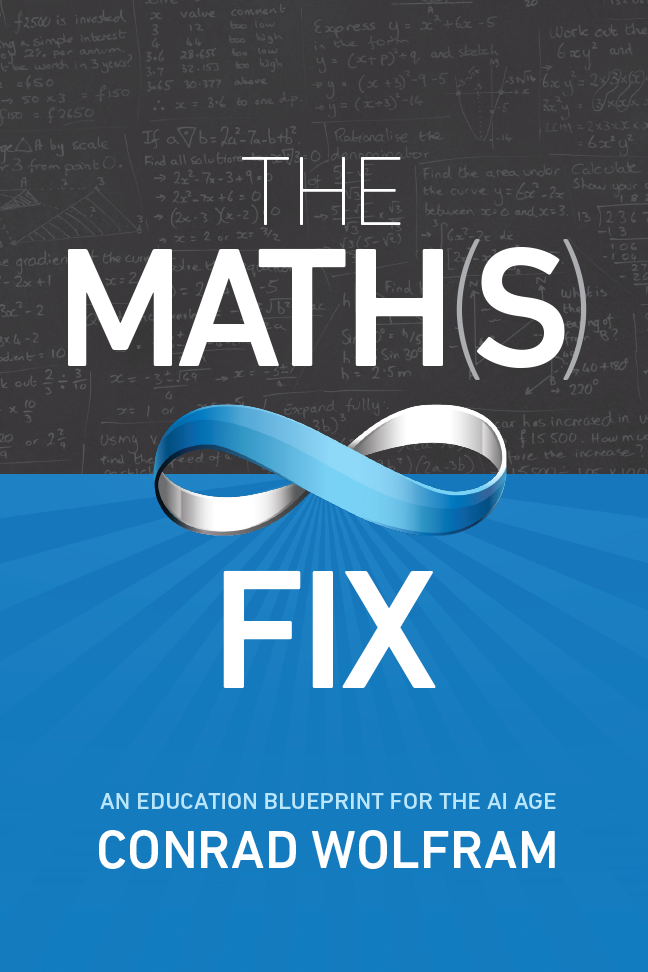## The Math(s) Fix

The Math(s) Fix: An Education Blueprint for the AI Age is a groundbreaking book that exposes why mathematics education is in crisis worldwide and how the only fix is a fundamentally new mainstream subject. Engaging and accessible yet deep and compelling, The Math(s) Fix argues that today’s math education isn’t working to elevate society with modern computation, data science and AI. Instead, students are subjugated to compete with what computers do best, and lose.

New books from other publishers include writings on advanced calculus, applied holography, quantum mechanics and more.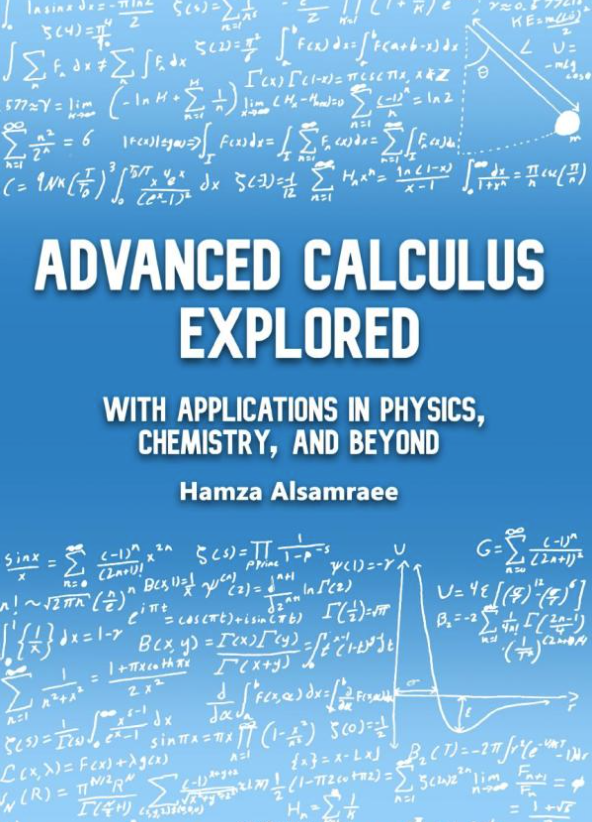## Advanced Calculus Explored: With Applications in Physics, Chemistry, and Beyond

Written by Wolfram Summer School participant Hamza Alsamraee, Advanced Calculus Explored gives readers tools for success in their STEM courses. The author’s use of the Wolfram Language to explore famous equations, applications in a range of topics and a multitude of nonstandard problems helps readers—especially students in advanced mathematics and science courses—build a stronger, more intuitive understanding of calculus.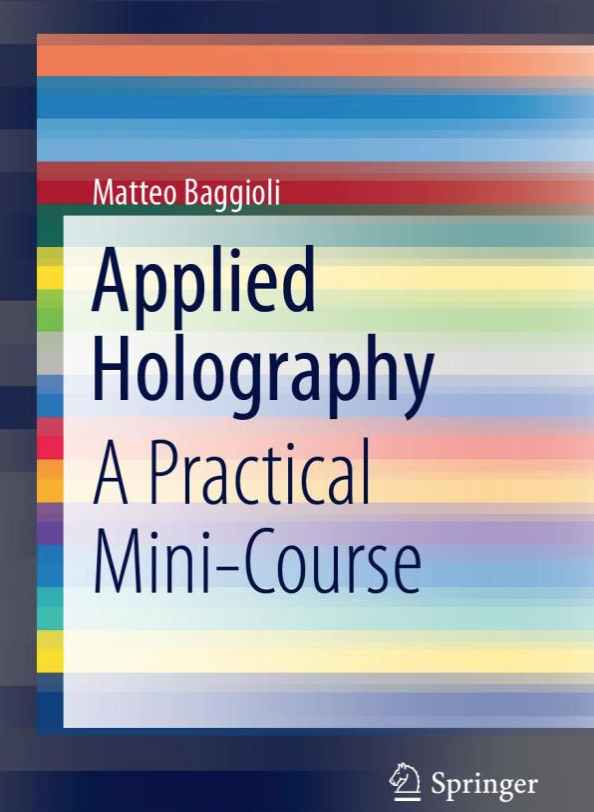## Applied Holography: A Practical Mini-Course

Originally presented as lectures given at the Indian Institute of Technology Madras (India) and at the Institute of Theoretical Physics Madrid (Spain), Matteo Baggioli’s new book is a concise and pragmatic course on applied holography. This primer focuses on analytic and numerical techniques, using Mathematica to detail computations and open-source numerical code. The author also shares tricks and techniques, supplementing concrete applications of AdS/CFT to hydrodynamics, quantum chromodynamics and condensed matter.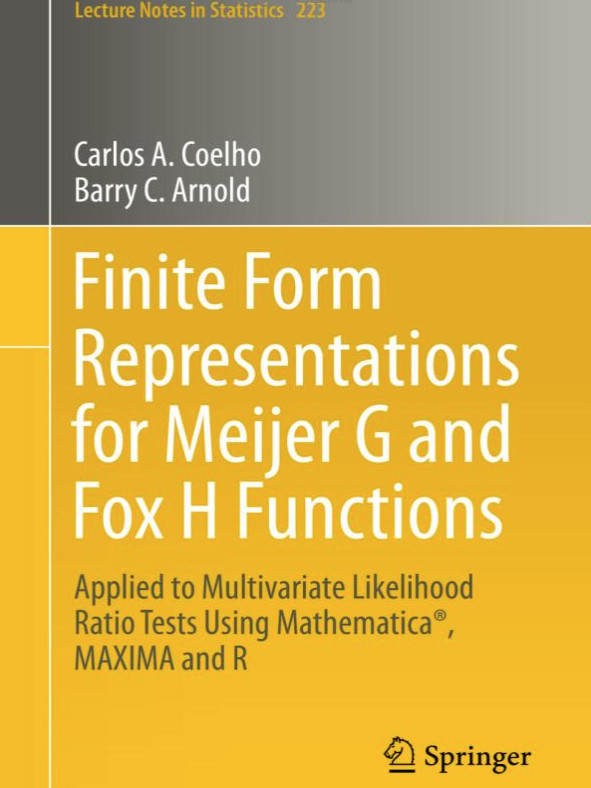## Finite Form Representations for Meijer G and Fox H Functions

Authors Carlos A. Coelho and Barry C. Arnold present computational modules in Mathematica and other languages to guide readers to implement, plot and compute the distributions of test statistics, or any other statistics that fit into the general paradigm described. Exact quantiles and the exact p-values of likelihood ratio tests can be computed quickly and efficiently by researchers and graduate students implementing likelihood ratio tests in multivariate analysis, providing an explicit manageable finite form for the distribution of the test statistics.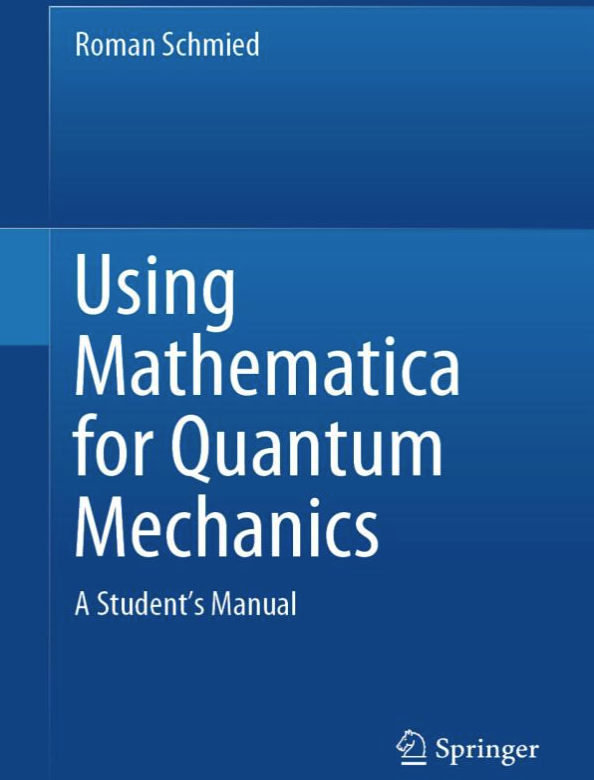## Using Mathematica for Quantum Mechanics: A Student’s Manual

Author Roman Schmied uses Mathematica to simulate many of the problems students encounter in introductory quantum mechanics. Computer implementations for finding and visualizing analytical and numerical solutions function as building blocks to solve more complex problems, such as coherent laser-driven dynamics in the Rubidium hyperfine structure or the Rashba interaction of an electron moving in 2D. This book is written in the Wolfram Language for its unparalleled ability to perform deep calculations, seamless mix of analytic and numerical facilities, built-in algorithms plus other libraries and the Wolfram Notebook interactive experience—but no prior knowledge of Mathematica is required.